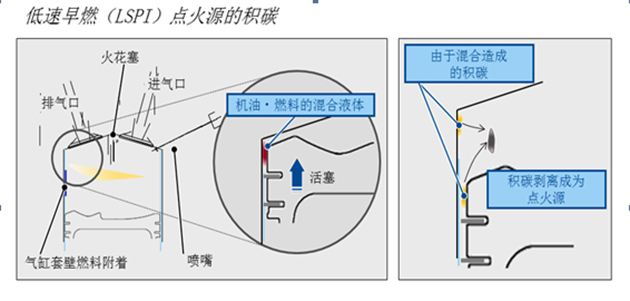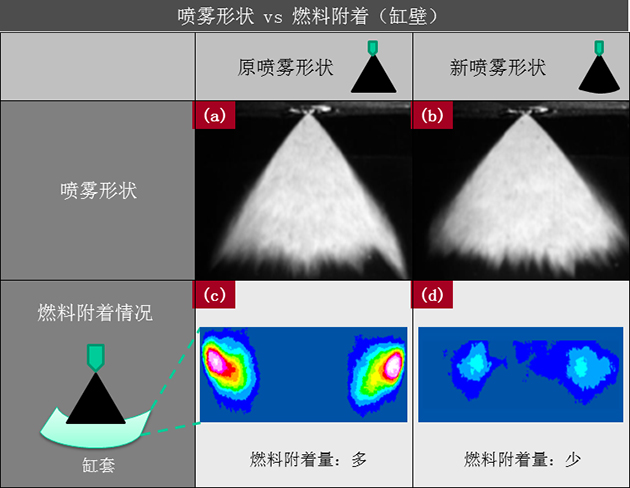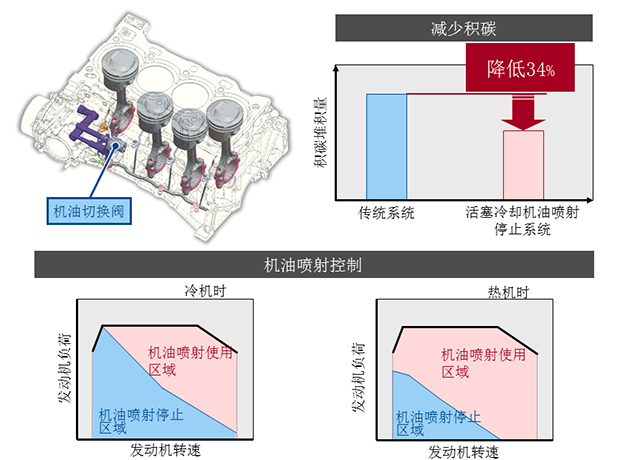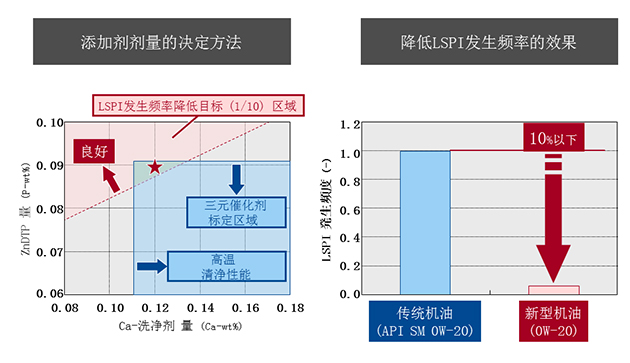## 史上最给力 全新皇冠不烧机油7个手段

2015年08月18日 15:00 来源：www.chextx.com 超过：次关注

提到涡轮增压发动机，很多人不免产生“烧机油”的担忧。事实上，每一款发动机都存在机油消耗的现象，不过由于涡轮增压发动机自身特点使其机油消耗相对于自然吸气发动机更高罢了，也就是人们常说的“烧机油”。因此，某些涡轮增压发动机被戏称为“混合动力”——（汽）油与机（油）混动型这样夸张也屡见不鲜。

那全新皇冠D-4ST 2.0T+发动机（8AR-FTS）在防止机油异常消耗上，采取了哪些措施呢？下面就让我们来看一看：

改良喷嘴形状

因为增压直喷发动机结构特点，燃油很容易沿着汽缸壁与活塞的间隙进入机油箱或者在活塞环缝隙生成燃料和机油堆积的混合物。燃油一旦进入机油箱势必会污染氧化机油；而活塞环缝隙间生成的混合物，一旦触碰到缸内被剥离的积碳则很容易被点燃，这也就是增压直喷发动机常遇到的低速早燃(LSPI)现象，而它会很轻松地加快机油的非正常消耗。正是基于此，丰田的设计师们将缸内直喷的喷油嘴设计成扁平形状并进一步加长，这样喷雾的形状与传统相比就更圆了，从而降低气缸壁上的燃料附着，截断、减少混合物的一部分来源。喷射时机

除了改良直喷喷嘴形状外，8AR-FTS发动机通过D-4ST双喷系统，对燃油喷射时机也进行了精心控制，在活塞吸气冲程和压缩冲程时均可喷射燃油，最大限度的提高混合效率，减少上述混合物与点火源——积碳的产生。

燃烧室形状

这款发动机在燃烧室上也做了不少文章，活塞顶部采用了特殊设计，提升了滚流比和混合气（汽油+空气）的流动强度，使分层燃烧和均质燃烧均可实现，进一步确保燃油的充分燃烧，降低早燃频率。

上述三项均是通过提高燃油效率，间接缓解机油非正常消耗的。那么8AR-FTS发动机又采取了哪些新技术、新手段来直接干涉机油损耗的呢？

机油喷射停止机构

丰田首次采用了对活塞冷却的机油喷射停止系统，该系统具有电子控制式切换阀，可依据发动机冷却液温度、发动机转速、负荷等，对机油喷射进行控制，可以降低机油的过度消耗与稀释。另外，这套系统也可降低发动机热机时活塞冠面的积碳堆积，而积碳的减少可为抑制低速早燃做贡献。曲轴通风系统

在曲轴通风系统中，丰田首次采用全新的喷射泵机构（新PCV系统），令自然吸气区域及增压区域曲轴内皆可实现通风，可以防止机油老化以及被进一步稀释。该系统的搭载，让8AR-FTS发动机的机油使用寿命与自然吸气发动机等同。

添加剂

除了创造外部“不烧机油”的环境，为了提升机油自身的抗氧化性以及耐久性，研发人员还为这款发动机添加了最优添加剂，开发出了新型机油。结构、工艺

8AR-FTS发动机采用了独一无二的设计结构，同时强化了活塞连杆；出于对零部件工艺、工程标准的苛求，整个发动机包括双涡管增压器均由丰田自制，从产品品质上保证了“不烧机油”。

从七种手段不难看出，为了走好重返涡轮增压领域的这一步，全新皇冠D-4ST 2.0T+发动机在可靠性上确实没少“下血本”，而且效果也不错。因为，到目前为止尚未有关于这款发动机（8AR-FTS已搭载在雷克萨斯品牌的部分车型上）“烧机油”的争论。此外，全新皇冠D-4ST 2.0T+车型即将上市，不用等太久，大家便可一睹这款发动机的四双（双喷射、双涡管、双循环、双VVT-i（W））、水冷技术、发动机启停节能技术等先进技术带来的“科技+、动力+、舒适+、可靠+”的完美表现，尤其是本文为大家详细阐述的惊世骇俗的“可靠+”。

#### 相关文章

﻿
• 快速找车
• 选择品牌
• 选择品牌
• A  奥迪
• A  阿斯顿·马丁
• A  阿尔法·罗密欧
• B  宝沃
• B  布加迪
• B  巴博斯
• B  保时捷
• B  宾利
• B  奔驰
• B  宝马
• B  本田
• B  别克
• B  标致
• B  比亚迪
• B  宝骏
• B  北汽制造
• B  北汽新能源
• B  北汽幻速
• B  北汽威旺
• B  北京汽车
• B  奔腾
• B  北汽绅宝
• B  北汽昌河
• C  长安欧尚
• C  长安
• C  长安凯程
• C  长城
• D  大众
• D  道奇
• D  DS
• D  东南
• D  东风风神
• D  东风风行
• D  东风小康
• D  东风风度
• D  东风
• F  福特
• F  丰田
• F  菲亚特
• F  法拉利
• F  福田
• F  福迪
• F  福汽启腾
• G  观致
• G  广汽传祺
• G  广汽吉奥
• G  GMC
• H  红旗
• H  汉腾汽车
• H  哈弗
• H  哈飞
• H  海格
• H  海马
• H  华颂
• H  黄海
• H  华泰
• H  恒天
• J  捷途
• J  几何汽车
• J  捷达
• J  吉利汽车
• J  捷豹
• J  Jeep
• J  江淮
• J  江铃
• J  金杯
• J  九龙
• J  金旅
• K  凯翼
• K  凯迪拉克
• K  克莱斯勒
• K  科尼塞克
• K  卡威
• K  开瑞
• L  路虎
• L  林肯
• L  劳斯莱斯
• L  兰博基尼
• L  雷克萨斯
• L  铃木
• L  领克
• L  雷诺
• L  理念
• L  力帆
• L  莲花汽车
• L  猎豹
• L  路特斯
• L  陆风
• M  马自达
• M  MG
• M  MINI
• M  玛莎拉蒂
• M  摩根
• M  迈凯轮
• N  纳智捷
• O  欧拉
• O  欧宝
• O  讴歌
• O  欧朗
• Q  奇瑞
• Q  起亚
• Q  启辰
• R  日产
• R  荣威
• R  瑞麒
• S  SERES赛力斯
• S  三菱
• S  斯威汽车
• S  萨博
• S  smart
• S  斯柯达
• S  斯巴鲁
• S  思铭
• S  双龙
• S  上汽大通
• S  双环
• T  特斯拉
• T  腾势
• W  蔚来
• W  沃尔沃
• W  WEY
• W  五菱汽车
• W  五十铃
• W  威兹曼
• W  威麟
• X  现代
• X  雪佛兰
• X  星途
• X  雪铁龙
• X  小鹏汽车
• X  西雅特
• Y  一汽
• Y  英菲尼迪
• Y  英致
• Y  依维柯
• Y  野马汽车
• Y  永源
• Z  众泰
• Z  中华
• Z  中兴
• Z  知豆
• 选择车系
• 选择车系
• 车型对比
• 选择品牌
• 选择品牌
• A  奥迪
• A  阿斯顿·马丁
• A  阿尔法·罗密欧
• B  宝沃
• B  布加迪
• B  巴博斯
• B  保时捷
• B  宾利
• B  奔驰
• B  宝马
• B  本田
• B  别克
• B  标致
• B  比亚迪
• B  宝骏
• B  北汽制造
• B  北汽新能源
• B  北汽幻速
• B  北汽威旺
• B  北京汽车
• B  奔腾
• B  北汽绅宝
• B  北汽昌河
• C  长安欧尚
• C  长安
• C  长安凯程
• C  长城
• D  大众
• D  道奇
• D  DS
• D  东南
• D  东风风神
• D  东风风行
• D  东风小康
• D  东风风度
• D  东风
• F  福特
• F  丰田
• F  菲亚特
• F  法拉利
• F  福田
• F  福迪
• F  福汽启腾
• G  观致
• G  广汽传祺
• G  广汽吉奥
• G  GMC
• H  红旗
• H  汉腾汽车
• H  哈弗
• H  哈飞
• H  海格
• H  海马
• H  华颂
• H  黄海
• H  华泰
• H  恒天
• J  捷途
• J  几何汽车
• J  捷达
• J  吉利汽车
• J  捷豹
• J  Jeep
• J  江淮
• J  江铃
• J  金杯
• J  九龙
• J  金旅
• K  凯翼
• K  凯迪拉克
• K  克莱斯勒
• K  科尼塞克
• K  卡威
• K  开瑞
• L  路虎
• L  林肯
• L  劳斯莱斯
• L  兰博基尼
• L  雷克萨斯
• L  铃木
• L  领克
• L  雷诺
• L  理念
• L  力帆
• L  莲花汽车
• L  猎豹
• L  路特斯
• L  陆风
• M  马自达
• M  MG
• M  MINI
• M  玛莎拉蒂
• M  摩根
• M  迈凯轮
• N  纳智捷
• O  欧拉
• O  欧宝
• O  讴歌
• O  欧朗
• Q  奇瑞
• Q  起亚
• Q  启辰
• R  日产
• R  荣威
• R  瑞麒
• S  SERES赛力斯
• S  三菱
• S  斯威汽车
• S  萨博
• S  smart
• S  斯柯达
• S  斯巴鲁
• S  思铭
• S  双龙
• S  上汽大通
• S  双环
• T  特斯拉
• T  腾势
• W  蔚来
• W  沃尔沃
• W  WEY
• W  五菱汽车
• W  五十铃
• W  威兹曼
• W  威麟
• X  现代
• X  雪佛兰
• X  星途
• X  雪铁龙
• X  小鹏汽车
• X  西雅特
• Y  一汽
• Y  英菲尼迪
• Y  英致
• Y  依维柯
• Y  野马汽车
• Y  永源
• Z  众泰
• Z  中华
• Z  中兴
• Z  知豆
• 选择车系
• 选择车系
• 选择车型
• 选择车型
• 意见反馈